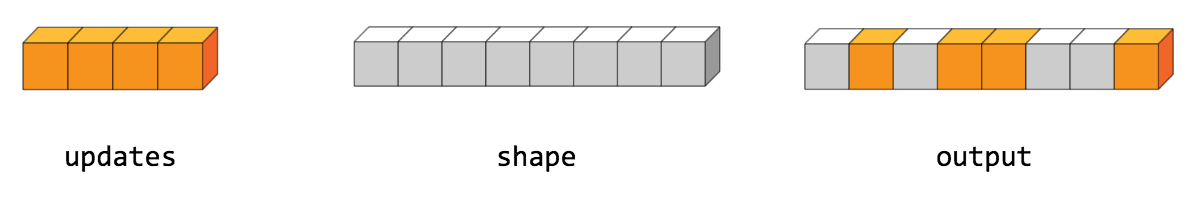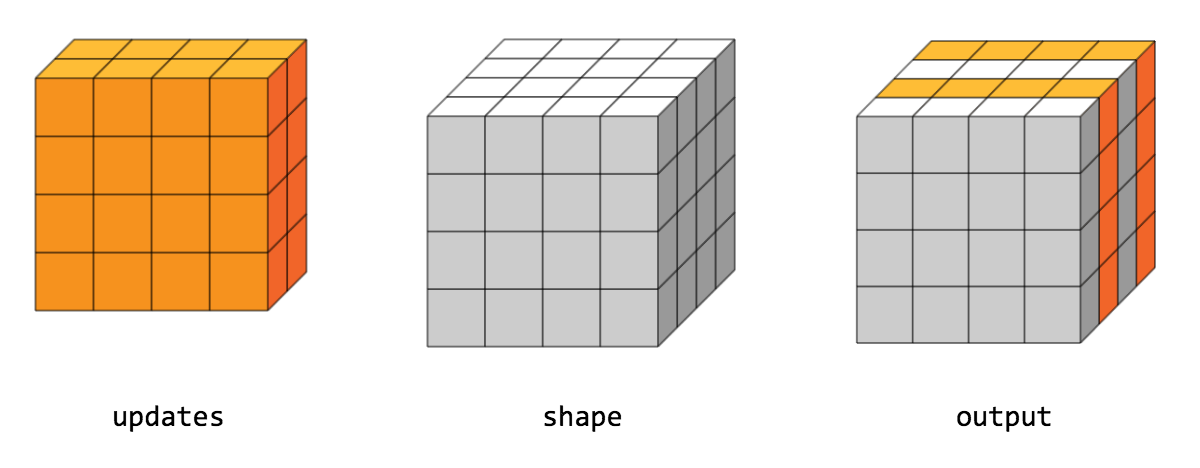Help protect the Great Barrier Reef with TensorFlow on Kaggle

ScatterNd

public final class ScatterNd

Scatter `updates` into a new tensor according to `indices`.

Creates a new tensor by applying sparse `updates` to individual values or slices within a tensor (initially zero for numeric, empty for string) of the given `shape` according to indices. This operator is the inverse of the `tf.gather_nd` operator which extracts values or slices from a given tensor.

This operation is similar to tensor_scatter_add, except that the tensor is zero-initialized. Calling `tf.scatter_nd(indices, values, shape)` is identical to `tensor_scatter_add(tf.zeros(shape, values.dtype), indices, values)`

If `indices` contains duplicates, then their updates are accumulated (summed).

WARNING : The order in which updates are applied is nondeterministic, so the output will be nondeterministic if `indices` contains duplicates -- because of some numerical approximation issues, numbers summed in different order may yield different results.

`indices` is an integer tensor containing indices into a new tensor of shape `shape`. The last dimension of `indices` can be at most the rank of `shape`:

indices.shape[-1] <= shape.rank

The last dimension of `indices` corresponds to indices into elements (if `indices.shape[-1] = shape.rank`) or slices (if `indices.shape[-1] < shape.rank`) along dimension `indices.shape[-1]` of `shape`. `updates` is a tensor with shape

indices.shape[:-1] + shape[indices.shape[-1]:]

The simplest form of scatter is to insert individual elements in a tensor by index. For example, say we want to insert 4 scattered elements in a rank-1 tensor with 8 elements.In Python, this scatter operation would look like this:

indices = tf.constant([, , , ])
updates = tf.constant([9, 10, 11, 12])
shape = tf.constant()
print(scatter)

The resulting tensor would look like this:

[0, 11, 0, 10, 9, 0, 0, 12]

We can also, insert entire slices of a higher rank tensor all at once. For example, if we wanted to insert two slices in the first dimension of a rank-3 tensor with two matrices of new values.In Python, this scatter operation would look like this:

indices = tf.constant([, ])
updates = tf.constant([[[5, 5, 5, 5], [6, 6, 6, 6],
[7, 7, 7, 7], [8, 8, 8, 8]],
[[5, 5, 5, 5], [6, 6, 6, 6],
[7, 7, 7, 7], [8, 8, 8, 8]]])
shape = tf.constant([4, 4, 4])
print(scatter)

The resulting tensor would look like this:

[[[5, 5, 5, 5], [6, 6, 6, 6], [7, 7, 7, 7], [8, 8, 8, 8]], [[0, 0, 0, 0], [0, 0, 0, 0], [0, 0, 0, 0], [0, 0, 0, 0]], [[5, 5, 5, 5], [6, 6, 6, 6], [7, 7, 7, 7], [8, 8, 8, 8]], [[0, 0, 0, 0], [0, 0, 0, 0], [0, 0, 0, 0], [0, 0, 0, 0]]]

Note that on CPU, if an out of bound index is found, an error is returned. On GPU, if an out of bound index is found, the index is ignored.

Public Methods

 Output () Returns the symbolic handle of a tensor. static ScatterNd ( Scope scope, Operand indices, Operand updates, Operand shape) Factory method to create a class wrapping a new ScatterNd operation. Output () A new tensor with the given shape and updates applied according to the indices.

Public Methods

public Output <U> asOutput ()

Returns the symbolic handle of a tensor.

Inputs to TensorFlow operations are outputs of another TensorFlow operation. This method is used to obtain a symbolic handle that represents the computation of the input.

public static ScatterNd <U> create ( Scope scope, Operand <T> indices, Operand <U> updates, Operand <T> shape)

Factory method to create a class wrapping a new ScatterNd operation.

Parameters
 scope current scope Index tensor. Updates to scatter into output. 1-D. The shape of the resulting tensor.
Returns
• a new instance of ScatterNd

public Output <U> output ()

A new tensor with the given shape and updates applied according to the indices.

[{ "type": "thumb-down", "id": "missingTheInformationINeed", "label":"Missing the information I need" },{ "type": "thumb-down", "id": "tooComplicatedTooManySteps", "label":"Too complicated / too many steps" },{ "type": "thumb-down", "id": "outOfDate", "label":"Out of date" },{ "type": "thumb-down", "id": "samplesCodeIssue", "label":"Samples / code issue" },{ "type": "thumb-down", "id": "otherDown", "label":"Other" }]
[{ "type": "thumb-up", "id": "easyToUnderstand", "label":"Easy to understand" },{ "type": "thumb-up", "id": "solvedMyProblem", "label":"Solved my problem" },{ "type": "thumb-up", "id": "otherUp", "label":"Other" }]